# DIY Project: How to Build Deck Stairs

Deck stairs may seem like a trivial project to complete, but without clear planning and precise measurements, the project may become quite expensive and take a long time to finish. Building deck stairs usually requires a lot of material regardless of whether it is wooden or concrete stairs. Usually, concrete stairs do not require as many measurements as wooden stairs because wooden stairs are composed of many different pieces that require precise measurements. The more measurements there are, the easier it is to buy material that does not fit the dimensions of the stairs. This article provides step-by-step guidance on how to plan and build deck stairs.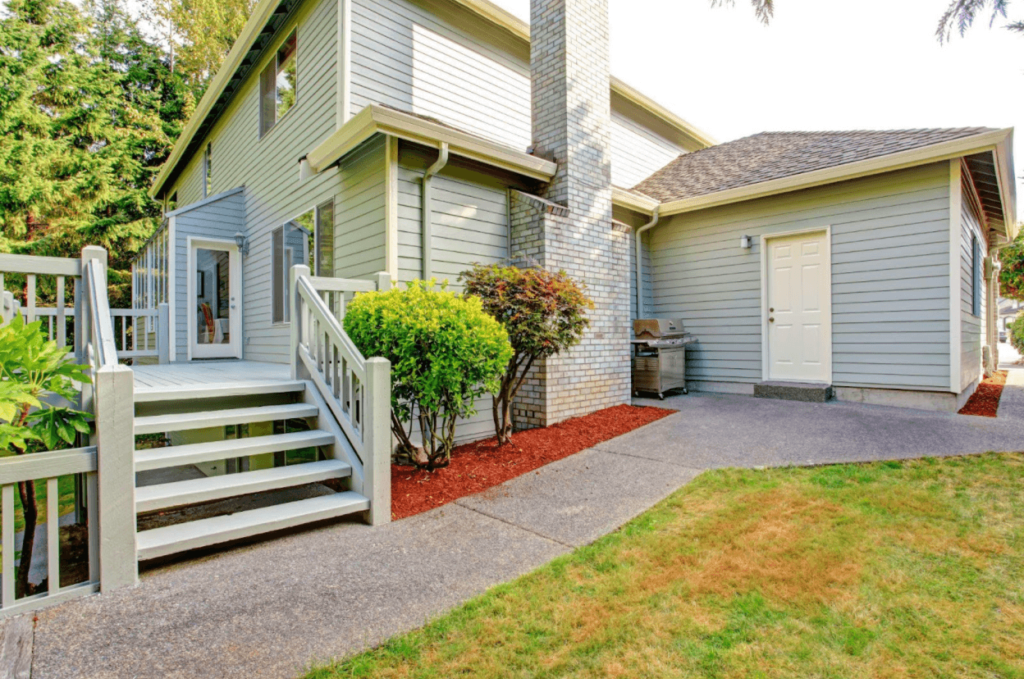# Step 1. Find the Necessary Dimensions

Before calculating the measurements for the deck stairs, you need to find three values that will determine the rest of the measurements needed to estimate the number of materials required to buy.

• Total Rise – the height of the deck.
• Total Run – length of deck stairs.
• Angle – the slope of the stairs.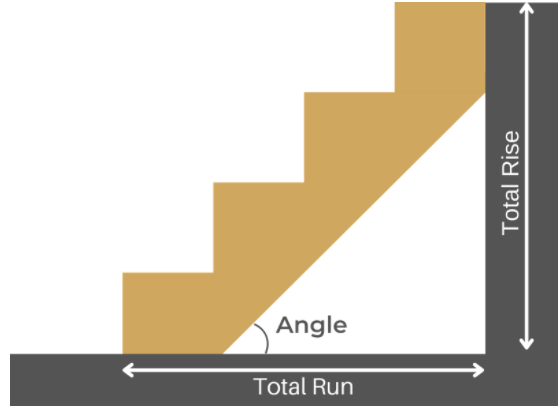The first step is to find the total rise of the stairs. The total rise of the stairs refers to the sum of the height of each stair. You can use a measuring tape to estimate the total rise of the stairs by placing one end of the tape at the ground and the other where the stairs end. You also need to know the total run of the stairs or the slope for the stairs. As a rule of thumb, the stairs should have a 40-degree angle slope. If you do not know the total run, you can approximate it by multiplying the total rise by 1.2. When you find the length, make sure you have enough space to place the stairs.

# Step 2. Calculate the Measurements

As soon as you know the dimensions of the space where the stairs will be placed, you can use the deck stair calculator. Given the total rise, you can calculate the number of steps the stair will have. An approximate height of one step is around 7 inches, so the number of steps can be approximated by dividing the total rise in inches by 7. If the result is a decimal, then rounding to the nearest whole number will solve the problem.

It is also important to decide whether deck stairs will have a flush mount or a standard mount. A flush mount and a standard mount are similar, but a slush mount has 1 stair less than a standard mount. In a flush mount, the deck plays the role of the missing stair while the stairs are attached to the deck. A standard mount, on the other hand, extends to the level of the deck.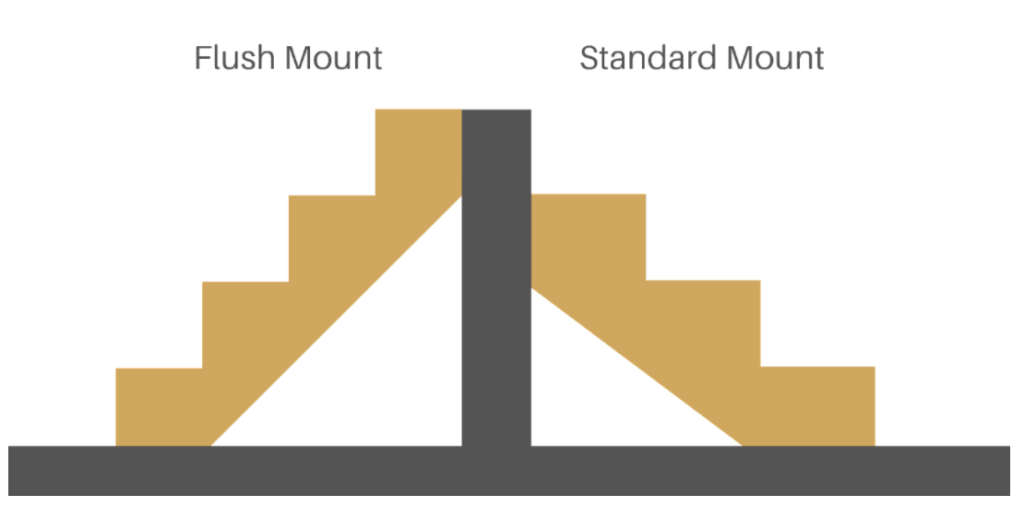The calculations for both types of stairs are very similar. The most basic wooden deck stairs require stringers, risers, and stair treads. All of these items require precise measurements to be built. The following process will help you find all the dimensions you need.

1. Find Rise and Run of a Single Stair

Knowing how many stairs you have, you can find the rise and run of a single stair for standard mount by dividing the total rise by the number of stairs and total run by the number of stairs minus one.

Rise = Total RiseNumber of Steps

Run = Total RunNumber of Steps – 1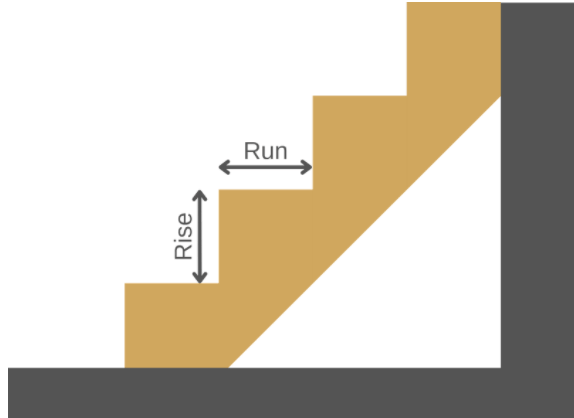If you decide to use a flush mount, then you should calculate the run of a single stair by dividing the total run by the number of steps.

Run = Total RunNumber of Steps

2. Calculate Stringer Dimensions

First, you need to calculate the height of the stringer. Height of the stringer is different for standard and flush mounts. Standard mount uses the deck as an extra step, so the height of a standard mount stringer is total rise minus one rise. Flush mount stringer extends all the way to the deck, so the height of a flush mount stringer is total rise.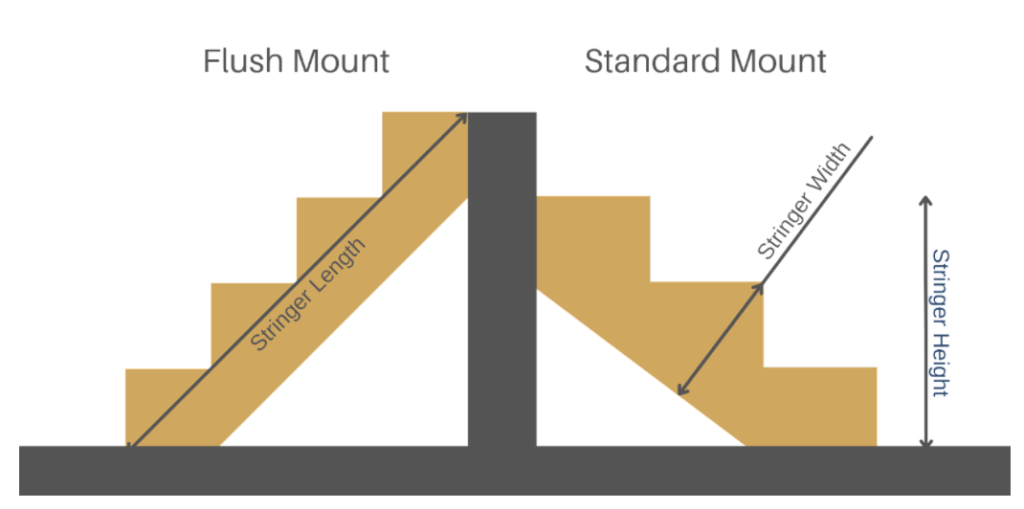The length of a stringer can be found using a Pythagorean theorem, stringer height and total run. The length of a stringer can be calculated as follows.

Stringer Length = Stringer Height2 + Total Run2

Width is another important metric that needs to be calculated. Width of the stringer depends on the specifics of the stairs, but as a rule of thumb, width of the stringer should be double the thickness of one step. Using this rule, you can calculate the width of the stringer by using the following formula.

Stringer Width = 2 Rise RunRise2 + Run2

With a stringer length and a stringer height, you can buy necessary material to make it.

3. Calculate Risers and Treads Dimensions

When you know the dimensions of the stringer, you need to find the length and width of risers and threads. Risers are placed vertically while threads are placed horizontally on a stringer to complete the stairs. The length of both risers and threads is equal to the width of the stairs. As a standard, stairs should be 3 feet wide, but the width may differ depending on the project.  When it comes to width, risers and treads have different widths, but it is very easy to find them. The width of a riser is equal to a rise of a single step, and the width of a tread is equal to a run of a single step.

4. Find the Elevation Angle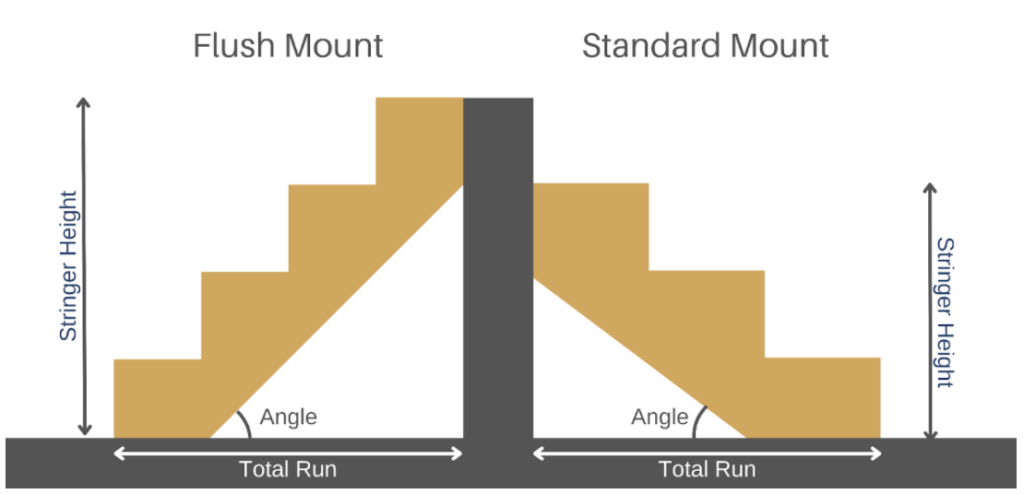Lastly, we need to find the exact elevation angle. This metric is important to make a stringer out of the wood planks that will fit the project specifications. You need to use the following formula to find the angle.

Angle = arctan(Stringer HeightTotal Run)

When you have all the necessary calculations ready, you can start working on the project.

# Step 3. Gather And Prepare Material

When you know the dimensions of the wooden planks you need and the number of stairs, you can go and find all the materials needed. In the case of standard wooden deck stairs, you will need two wooden planks for a stringer, a number of risers equal to the number of steps, and the number of threads equal to the number of steps minus one. It is wise to get 10% extra material for cases when errors are made and more material is needed.

When you have the planks ready, you should clearly draw what parts need to be cut. A square is a useful measuring tool in this situation because it can be used to draw the stairs on a stringer easily. If you did not buy risers and treads and purchased wood planks instead, you should mark their length and width to cut them properly. To make sure that no mistakes are made, you can fit the plank where it should be and see whether the marking is correct. When all the material is clearly marked, you can cut off the unwanted parts. You might want to consider protecting the wood by painting it or applying wood oil to it.

# Step 4. Assemble the Stairs

Make sure that the landing point is solid. The landline point is where the stairs meet the ground. When you have the material ready, you can finally assemble the stairs. You can start by fitting the stringer. If it is not stable at the landing area, you may need to adjust the end of the stringer for it to be stable. When the stringer planks are attached to the deck and are stable on the ground, you can attach the risers and the treads. It is better to attach the risers before the treads because if a tread is not properly installed, then a riser may not fit.

When it is installed, you can compare the result with the measurements to make sure that the deck stairs are built correctly. This project requires a certain amount of skill in construction, so an inexperienced person should ask for help when building a deck staircase.